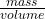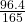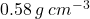## 4) A piece of wood in the shape of a rectangular prism has dimensions of 11 cm by 5 cm by 3 cm. It has a mass of 96.4 grams Datormine

Question

4) A piece of wood in the shape of a rectangular prism has dimensions of 11 cm by 5 cm by 3 cm.
It has a mass of 96.4 grams Datormine its density.

in progress 0
2 weeks 2021-09-04T11:07:54+00:00 1 Answers 0 views 0

density = 0.58 g/cm^3

Step-by-step explanation:

• Mass = 96.4 gram

Volume of rectangular prism = L × B × H

( Where, L = length, B = width, H = height )

=》11 × 5 × 3

=》165 cubic cm

• volume = 165 cubic cm

Now, we know that density ==》=》density = 0.58 grams per centimeter cube

( g / cm^3)